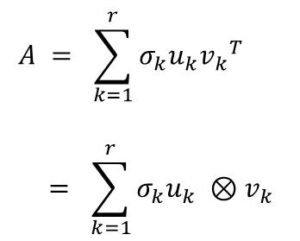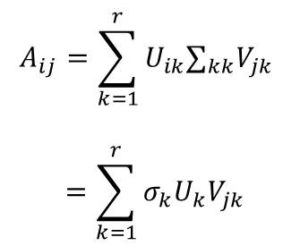# Introduction to Higher Order Singular Value Decomposition (HOSVD)

I would start with a brief review of SVD and then gradually ease into Higher Order SVD.  As usual, I would try to make it as easy and clear as possible.1. Review of Singular Value Decomposition(SVD)
3. What is Higher Order SVD (HOSVD)
4. Basic Concepts of HOSVD
5. The HOSVD Algorithm

Review of Singular Value Decomposition (SVD)

Recall that Singular Value decomposition is a technique to decompose a data matrix into three parts.

Given a rectangular matrix A which is an n x p matrix, the SVD theorem shows that the matrix can  be represented as:

A = U∑VT(same as  U∑V*)

where

• A is the original data matrix  of size m x n
• U is  the left singular vectors of size m x r
• ∑  is the  singular values on its diagonal of size r x r
• V* is right singular vectors of size r x n

Now we are going to represent the SVD still in a slightly different way. This time as as summation of different components:In this case the, symbol ⊗ represents the tensor product (remember that a matrix is also a tensor, but of rank 0). This means that each element of a can be represented in this way:As like before,

• Σ is a diagonal r x r matrix with non-zero singular values in its diagonal
• σ are the actual singular values while
• uk are the columns of the matrix U (m x r)
• vk are the columns of the matrix V (r x n)

This is illustrated in Figure 1a and Figure 1b shown below. Keep in mind that we are still doing a review in SVD, but trying to generalize as this would make understanding of Higher Order SVD easier to follow.

Figure 1a and Figure 1b are approximate to the same. In 1 b, we are taking summation over the the product of the slices of the columns of U with slices of  rows of VT. Also not that we are dotting each term with the corresponding singular value.

Now, we are set to now consider Higher Order SVD.  The Singular Value Decomposition(SVD) can be generalized to higher order tensors or multi-way arrays in different ways.

In this article, we are going to consider two main approaches, namely:

1. Tucker decomposition (HoSVD decomposition)
2. CP Expansion (Canonical decomposition/Parallel facotorisation)

We would continue with these in the next article. Do check in couple of days.#### kindsonthegenius

Kindson Munonye is currently completing his doctoral program in Software Engineering in Budapest University of Technology and Economics

View all posts by kindsonthegenius →